## Beyond the Textbook

These tutorials are covering practical applications of image processing algorithms, tomography and machine learning.

Free resources for coding enthusiasts.

#### Pydingo neutron CT reconstruction software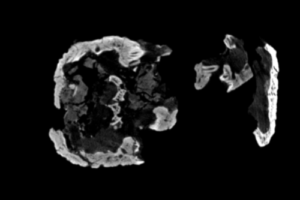A short introduction to our neutron CT reconstruction software developed for the Dingo instrument at the Australian Centre for Neutron Scattering (ANSTO).

#### Comparison of tomography reconstruction techniques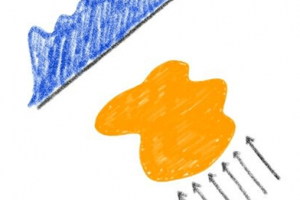An overview of different types of tomography reconstruction algorithms, their strengths and pitfalls when it comes to reconstructing tomography data.

#### 3D network graphs with Python and the mplot3d toolkitA worked example of a 3D network graph using Networkx and the mplot3d toolkit of Matplotlib in Python.

#### Interactive choropleth maps with Plotly for PythonLearn how to make stunning interactive choropleth maps using Plotly for Python.

#### Revealing interactive scatter plots with Plotly for Python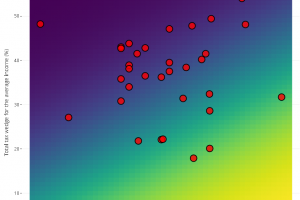Scatter plots are very powerful at visualising correlations of 2D data. Learn how to build a revealing interactive scatter plots with Plotly for Python.

#### Animated 3D graphs with Matplotlib mplot3d toolkitA worked example of a simple animated 3D scatter plot using the mplot3d toolkit of Matplotlib in Python.

#### GPU accelerated FFT compatible with Numpy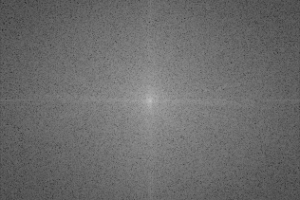GPU accelerated FFT and IFFT functions using Python and pycuda, designed to be fully compatible with the corresponding Numpy functions.

#### Unsharp masking with Python and OpenCV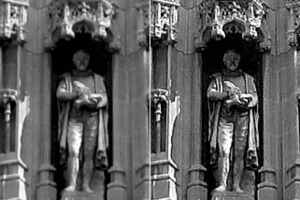How does unsharp masking work? And how we can sharpen or blur images? Here’s a short tutorial on unsharp masking with Python and OpenCV.

#### Time-lapse photography with Python and OpenCV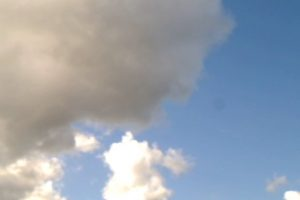A simple example of time-lapse photography using a USB camera driven by OpenCV in Python.

#### Quantitative porosity analysis of volumetric data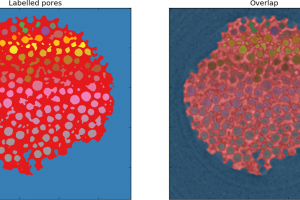Extract the quantitative porosity distribution from volumetric data of porous samples.

#### Segmentation using k-means clustering in PythonSegmentation is used for feature extraction in images and volumes. In this post we discuss how to segment an image using k-means clustering.